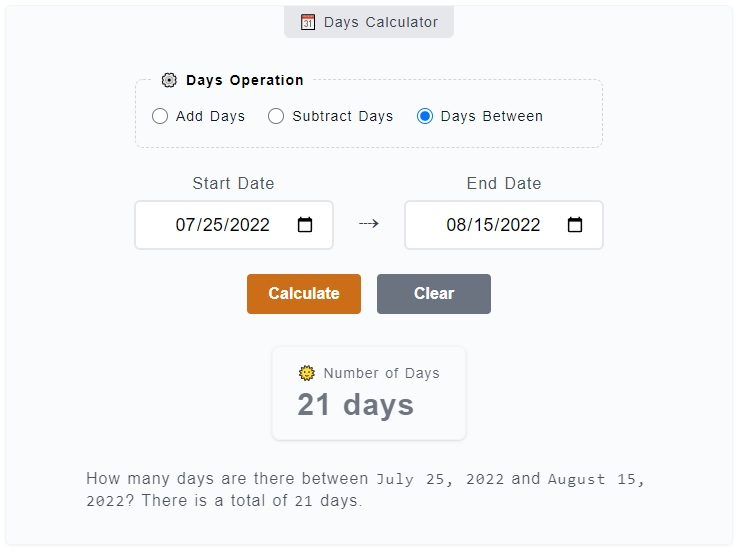# Days Calculator

The Days Calculator is primarily used to get the days between two dates but you can also add and subtract days from a date. For example, you want to know how many days are there in between and ? Just enter them on the calculator. You can also add `2 days` or subtract `21` days using this calculator.

🗓️ Days Calculator
⚙️ Days Operation

### 🌞 Number of Days

21 days

How many days are there between `June 23, 2023` and `July 14, 2023`? There is a total of `21` days.

## How to Use the Days Calculator

To use the Days Calculator, you just need to pick first the operation you want to use - add days, subtract days or get the days between two dates. You just need to enter the values on corresponding inputs and click on `Calculate` button. Here's a more detailed step by step guide.1. Step 1

Above the main calculator, you can find the `⚙️ Days Operation` field set. It is where you need to choose from the operation options - add or subtract days and get the days between. Let's an example here, please select the `days between` option which is already selected by default.

2. Step 2

After you have chosen an operation type, the next thing to do is to fill the input fields - `Start Date` and `End Date`. The Start Date represents the base or reference date of the count-off while the End Date is where the count-off ends.

3. Step 4

The final step is to click on the `Calculate` button to get your results. You can reset the filled input fields as well by just clicking on the `Clear` button.

In case you are doing some addition or subtraction of days from the starting date, first select the `add` or `subtract` as the type of operation. Then, enter the starting date and the number of days want to add or subtract on their corresponding fields.

Although we have a much specific calculator for days from now (➕ add days) and days ago (➖ subtract days), this calculator has more option to change the starting date and combined with days between two dates operation.